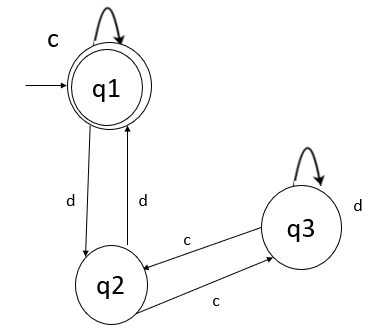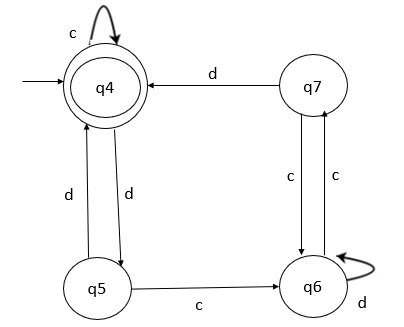# Explain the equivalence between two Finite State Machines.

The two finite automata (FA) are said to be equivalent if both the automata accept the same set of strings over an input set Σ.

When two FA’s are equivalent then, there is some string x over Σ. On acceptance of that string, if one FA reaches to the final state, the other FA also reaches to the final state.

## Method

The method for comparing two FA’s is explained below −

Let M and M1 be the two FA’s and Σ be a set of input strings.

Step 1 − Construct a transition table that has pairwise entries (q, q1) where q ∈ M and q1 ∈ M1 for each input symbol.

Step 2 − If we get in a pair as one final state and other non-final state then we terminate the construction of transition table declaring that two FA’s are not equivalent

Step 3 − The construction of the transition table gets terminated when there is no new pair appearing in the transition table.

## Example

Consider the two Deterministic Finite Automata (DFA) and check whether they are equivalent or not.## Explanation

• Step 1 − First, construct the transition table for each input c and d.
• Step 2 − From the first machine M on receiving input c in state q1, we reach state q1 only which is the final state.
• Step 3 − From the second machine M1 for state q4 on receiving input c, we reach state q4 which is the final state.
• Step 4 − Thus for state (q1, q4) for input c, we get the next states as (q1, q4). Both are final states.
• Step 5 − Similarly, for input d in state (q1, q4), we get the next state as (q2, q5). Both are intermediate states. So, the first state in both machines is equal.
• Step 6 − Similarly, we perform the remaining states in two machines.
• Step 7 − In a pair of states, if both are final or if both are non-final, then we can say that two DFA’s are equivalent and let’s check for remaining.

The transition table is as follows −

Statescd
{q1,q4}{q1, q4}
FS FS
{q2, q5}
IS IS
{q2,q5}{q3, q6}
IS IS
{q1, q4}
FS FS
{q3, q6}{q2, q7}
IS IS
{q3, q6}
IS IS
{q2, q7}{q3, q6}
IS IS
{q1, q4}
FS FS

Here, FS is the Final State and IS is the Intermediate State.

Therefore, by seeing the above table it is clear that we don’t get one final and one intermediate in any pair. Hence, we can declare that two DFA’s are equivalent.